﻿ Piecewise-Defined Functions

# PIECEWISE-DEFINED FUNCTIONS

• PRACTICE (online exercises and printable worksheets)

A piecewise-defined function has different rules for different inputs.

Here is a typical piecewise-defined function:
 $$f(x) = \cases { -x + 3 &\text{if }\quad -1 \le x < 1\cr 5 &\text{if }\quad 1 \le x < 2\cr x^2 &\text{if }\quad x\ge 2 }$$ or, with slightly different formatting and notation: $$f(x) = \cases { -x + 3\,, &\text{if }\quad x\in [-1,1)\cr 5\,, &\text{if }\quad x\in [1,2)\cr x^2\,, &\text{if }\quad x\in [2,\infty) }$$

Recall that ‘$\,x\in [-1,1)\,$’ means that $\,x\,$ is a member of the interval $\,[-1,1)\,$.

## Finding Function Values for a Piecewise-Defined Function

This function $\,f\,$ uses three rules (one for each row)—that is, it has three ‘pieces’ (hence the name piecewise-defined function):
• for inputs in the interval   $\,[-1,1)\,$   the linear function   $\,-x+3\,$   is used for the outputs
• for inputs in the interval   $\,[1,2)\,$   the constant function   $\,5\,$   is used for the outputs
• for inputs in the interval   $\,[2,\infty)\,$   the quadratic function   $\,x^2\,$   is used for the outputs
Here are some examples:

find this function value Which interval is $\,x\,$ in? so, use this rule... solution
$f(-1)$ $-1\in [-1,1)$ $-x+3\,$   (first row) $f(-1) = -(-1) + 3 = 4$
$f(0)$ $0\in [-1,1)$ $-x+3\,$   (first row) $f(0) = -(0) + 3 = 3$
$f(0.9)$ $0.9\in[-1,1)$ $-x+3\,$   (first row) $f(0.9) = -(0.9) + 3 = 2.1$
$f(1)$ $1\in [1,2)\,$ $5\,$   (second row) $f(1) = 5$
$f(1.99999)$ $1.99999 \in [1,2)$ $5\,$   (second row) $f(1.99999) = 5$
$f(2)$ $2 \in [2,\infty)$ $x^2\,$   (third row) $f(2) = 2^2 = 4$
$f(\pi)$ $\pi \in [2,\infty)$ $x^2\,$   (third row) $f(\pi) = \pi^2$

## Reading a Piecewise-Defined Function Aloud

If forced to read the function     $\displaystyle f(x) = \cases { -x + 3 &\text{if }\quad -1 \le x < 1\cr 5 &\text{if }\quad 1 \le x < 2\cr x^2 &\text{if }\quad x\ge 2 }$     aloud, it is typically read from left-to-right and top-to-bottom.
One possible reading could go like this:

Eff of ex equals negative ex plus three, if ex is greater than or equal to negative one and less than one;
it equals five, if ex is greater than or equal to one and less than two;
it equals ex squared, if ex is greater than or equal to two.

More often, though, people working with such a formula will just look at it and use it!

Here are additional things you should be able to do with a piecewise-defined function:

Finally, you should be aware of variations in notation for piecewise-defined functions.

## Finding the Domain of a Piecewise-Defined function

The domain is easy to get from the formula alone.
Just ‘put together’ (union) the input sets from all the rows.
Combined, these are the values of $\,x\,$ that $\,f\,$ knows how to act on. $$f(x) = \cases { -x + 3 &\text{if }\quad -1 \le x < 1\cr 5 &\text{if }\quad 1 \le x < 2\cr x^2 &\text{if }\quad x\ge 2 }$$

 top row $[-1,1)$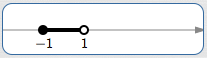second row $\,[1,2)\,$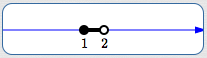third row $\,[2,\infty)\,$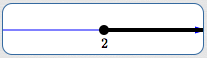union, to get the domain of $\,f\,$ $\text{dom}(f) = [-1,\infty)\,$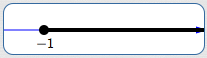By the way, the range of $\,f\,$ is nowhere near as easy to get from the formula.
Once you have a graph, though, the range is easy to obtain:
just use the ‘collapse the graph into the $\,y\,$-axis’ method, as described in this earlier lesson.

## Graphing a Piecewise-Defined Function

When a graph is ‘pieced together’ using different rules, as in a piecewise-defined function,
you may need up to three ‘dots’ to fully understand what's happening at a place where rules are changing.
This is illustrated in the sketch below:In this example, three rules are needed to fully understand what happens at and near $\,x\,$: There is a rule that applies at $\,x\,$. This produces the solid (filled-in) dot, with coordinates $\,\bigl(x,f(x)\bigr)\,$. This is an actual point on the graph of the function; it is an (input,output) pair. There is a rule that applies to the left of $\,x\,$. The hollow dot at $\,\color{red}{(x,\ell)}\,$ is NOT a point on the graph; it is NOT an (input,output) pair. When the input is $\,x\,$, the output is NOT $\,\ell\,$! Instead, this hollow dot is used to show limiting behavior. It answers the question: What happens to the outputs as the inputs approach $\,x\,$ from the left-hand side? (Answer: the outputs approach the number $\,\ell\,$) There is a rule that applies to the right of $\,x\,$. The hollow dot at $\,\color{green}{(x,r)}\,$ is NOT a point on the graph; it is NOT an (input,output) pair. When the input is $\,x\,$, the output is NOT $\,r\,$! Instead, this hollow dot is used to show limiting behavior. It answers the question: What happens to the outputs as the inputs approach $\,x\,$ from the right-hand side? (Answer: the outputs approach the number $\,r\,$) Remember: for a given value of $\,x\,$, there can be at most one solid dot; to be a function, each allowable input must have exactly one output.

Next, we graph: $$f(x) = \cases { -x + 3 &\text{if }\quad -1 \le x < 1\cr 5 &\text{if }\quad 1 \le x < 2\cr x^2 &\text{if }\quad x\ge 2 }$$ The ‘pieces’ may be graphed in any order.
Here, the choice is made to move from left-to-right on the number line (from top-to-bottom in the formula).

On $\color{red}{[-1,1)}$, $\,f\,$ is linear, since $\,\color{red}{-x+3}\,$ is of the form $\,mx + b\,$.

The endpoint $\,-1\,$ is included in the interval, indicated by the use of the bracket ‘$\,[\,$’ in interval notation.
The endpoint $\,1\,$ is excluded from the interval, indicated by the use of the parenthesis ‘$\,)\,$’ in interval notation.

$x$ is ... included or excluded? substitute into $\,-x+3\,$ plot the point ... using this kind of dot
$-1$ included $-(-1) + 3 = 1 + 3 = 4$ $(-1,4)$ solid
$1$ excluded $-(1) + 3 = -1 + 3 = 2$ $(1,2)$ hollow

Important: the point $\,(1,2)\,$ is not a point on the graph of $\,f\,$.
This hollow dot is used to show limiting behavior:
as the inputs approach $\,1\,$ from the left-hand side, the outputs approach $\,2\,$.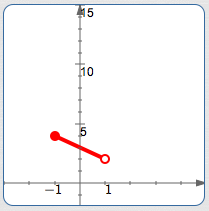On $\color{blue}{[1,2)}$, $\,f\,$ is constant with value $\color{blue}{5}$, so it graphs as a horizontal segment.

$x$ is ... included or excluded? substitute into
the constant function $5$
plot the point ... using this kind of dot
$1$ included $5$ $(1,5)$ solid
$2$ excluded $5$ $(2,5)$ hollow

Important: the point $\,(2,5)\,$ is not a point on the graph of $\,f\,$.
This hollow dot is used to show limiting behavior:
as the inputs approach $\,2\,$ from the left-hand side, the outputs approach $\,5\,$.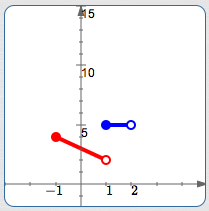On $\color{green}{[2,\infty)}$, $\,f\,$ is quadratic,since $\,\color{green}{x^2}\,$ is of the form $\,ax^2 + bx + c\,$.
Thus, it graphs as a piece of a parabola on this interval.

Think about what the entire graph of $\,y = x^2\,$ looks like (it is lightly dashed here),
and draw in the portion for the inputs $[2,\infty)\,$.

$x$ is ... included or excluded? substitute into $\,x^2$ plot the point ... using this kind of dot
$2$ included $2^2 = 4$ $(2,4)$ solidHere is the graph of the piecewise-defined function $\,f\,$, color-coded with its graph: $$f(x) = \cases { \color{red}{-x + 3} &\text{if }\quad -1 \le x < 1\cr \color{blue}{5} &\text{if }\quad 1 \le x < 2\cr \color{green}{x^2} &\text{if }\quad x\ge 2 }$$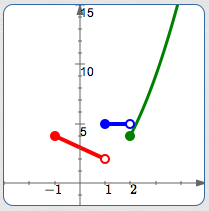## Getting the Formula for a Piecewise-Defined Function from its Graph

To go from a graph to a formula, you need additional information for any non-linear and non-constant sections, perhaps like this:There will be three rows in the piecewise-defined function, since there are three ‘pieces’.

You may want to put the proper inputs in place first;
left-to-right on the number line corresponds to top-to-bottom in the rows.
Be very careful about solid dots and hollow dots!

Put any ‘easy’ formulas in place at the same time (like constant functions and labeled pieces): $$f(x) = \cases { ... &\text{if }\quad -1 \le x < 1\cr 5 &\text{if }\quad 1 \le x < 2\cr x^2 &\text{if }\quad x\ge 2 }$$

It remains only to get the formula for the linear section:

• read off the coordinates $\,(-1,4)\,$ and $\,(1,2)\,$
• find the slope:   $m = \frac{2-4}{1 - (-1)} = \frac{-2}{2} = -1$
• use point-slope form with either endpoint:   $y - 2 = -(x - 1)$
• solve for $\,y\,$:   $y = -(x-1) + 2 = -x+1+2 = -x+3$
Put the formula $\,-x+3\,$ in the top row. Done!

## Variations in Notation for Piecewise-Defined Functions

There are a variety of styles for formatting piecewise-defined functions, including (but not limited to):
• using the word ‘for’ instead of ‘if’ to specify the input values
• putting commas after each rule
• putting commas at the very end of all rows but the last
Lots of different combinations are possible. Here are some examples:
 $$f(x) = \cases { -x + 3\color{red}{\,,} &\text{if }\quad -1 \le x < 1\cr 5\color{red}{\,,} &\text{if }\quad 1 \le x < 2\cr x^2\color{red}{\,,} &\text{if }\quad x\ge 2 }$$ (commas after each rule) $$f(x) = \cases { -x + 3\,, &\text{if }\quad -1 \le x < 1\color{green}{\,,}\cr 5\,, &\text{if }\quad 1 \le x < 2\color{green}{\,,}\cr x^2\,, &\text{if }\quad x\ge 2 }$$ (commas at the very end of all rows but the last) $$f(x) = \cases { -x + 3\,, &\text{for}\quad -1 \le x < 1\cr 5\,, &\text{for}\quad 1 \le x < 2\cr x^2\,, &\text{for}\quad x\ge 2 }$$ (use the word ‘for’ instead of ‘if’)

With beautiful typesetting (including all the words ‘ if ’ lined up vertically), this author prefers the simplest formatting, with no extra punctuation.

Master the ideas from this section